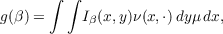#### Vol. 30, No. 3, 1969

 Download this articleFor screen For printingRecent Issues Vol. 325: 1 Vol. 324: 1  2 Vol. 323: 1  2 Vol. 322: 1  2 Vol. 321: 1  2 Vol. 320: 1  2 Vol. 319: 1  2 Vol. 318: 1  2Online Archive Volume: Issue:The Journal Subscriptions Editorial Board Officers Contacts Submission Guidelines Submission Form Policies for Authors ISSN: 1945-5844 (e-only) ISSN: 0030-8730 (print) Special Issues Author Index To Appear Other MSP Journals
Measures on countable product spaces

### Edwin O. Elliott

Vol. 30 (1969), No. 3, 639–644
##### Abstract

A regular conditional measure ν on a space Y relative to an outer measure μ on a space X is defined as a function on X ×ℛ such that (1) for each x X,ν(x,) is an outer measure on Y and is the family of subsets of Y which are (Carathéodory) measurable under each of the measures ν(x,),x X, and (2) for each β ∈ℛ the function ν() on X is μ integrable) i.e., ν(x,β)μdx .

Letting g be the function on the subsets of Z = X × Y defined bydefining a covering family F to consist of those rectangles A × B where A is μ measurable, B ∈ℛ and g(A×B) < or those sets N such that g(N) = 0, we obtain the outer measure ϕ = (μν) on Z generated by (the content) g and covering family F.

A system of regular conditional measures is a sequence begun by a measure ν0 on a space X1 and followed by regular conditional measures νi (relative to μi) on spaces Xi+1(i = 1,2,) where μ1 = ν0 and μi+1 = (μi νi) for i = 1,2, . Set X = xXi, and for x X write xi for the point (x1,x2,,xi) which is the projection of x onto the space Xi = j=1iXj and similarly write Si = j=1iSj whenever the sets Sj are subsets of Xj(j = 1,,i).

For such a system of regular conditional measures a generalization of Tulcea’s extension theorem for regular conditional probabilities holds, a Fubini-like theorem for integrable functions is obtained and finally, for topological spaces, a condition is given for the extension of inner regularity and almost Lindelöfness properties.

Primary: 28.40
##### Milestones